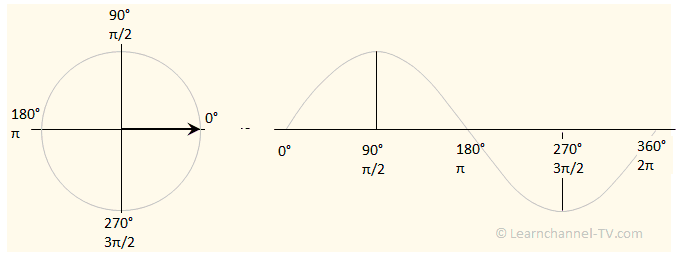### Sinusoidal Waveform Construction

Sinusoidal alternating variables can be displayed both in a line chart or as a pointer diagram. In the pointer diagram, the pointer rotates counterclockwise. The pointer length corresponds to the peak value of the alternating quantity.Sinusoidal Waveform Construction

For a given peak value up , the instantaneous value u(t) of a sinusoidal alternating voltage can be calculated for any point in time or angle:

u(t) = f(α) = up  sin(α)                                  (1)

In the pointer diagram, the pointer with the length r travels the distance s within one period, which corresponds to the circumference :

s = 2 π r                                                     (2)

The period duration is directly related to the rotational speed:

v = (2 π r)    because  t = T                          (3)
T

In order to be independent of the radius length, we now refer to the unit circle with r = 1 and thus get the angular velocity ω with the unit s-1:

ω =  v   = ( 2 π )         f =  1 / T           =>           ω = 2 π f          (4)
.        r           T

The angular velocity ω is the angular change per time.

The angle passed in a given time is determined by the following equation:

α = ω t = 2 π f  t                                                                             (5)

If the peak value and frequency also are known, then the instantaneous value can be determined for each point in time:

u = f(t) = up * sin(ω t) = up * sin(2 π f  t)                                 (6)